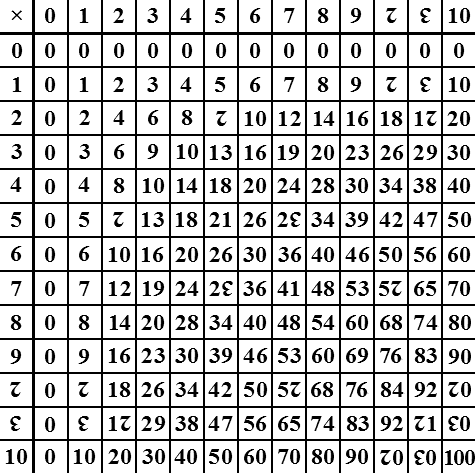Definition of

# DuodecimalNumbers based on 12 digits.

The digits are: 0, 1, 2, 3, 4, 5, 6, 7, 8, 9, A and B.
(But some people use other symbols instead of A and B, such as an upside-down 2 and 3.)

Example Duodecimal Numbers:
• The decimal number 10 is A
• The decimal number 11 is B
• The decimal number 12 is 10
• The decimal number 13 is 11
• The decimal number 50 is 42

Also called "dozenal", because 12 is a dozen.

Below is a dozenal multiplication table: